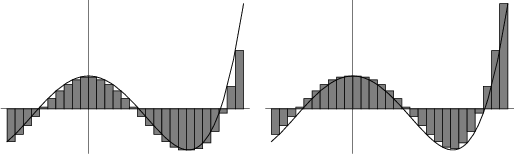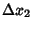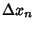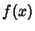## Riemann SumLet a Closed Intervalbe partitioned by points, the lengths of the resulting intervals between the points are denoted,, ...,. Then the quantityis called a Riemann sum for a given functionand partition. The valueis called the Mesh Size of the partition. If the Limitexists, this limit is known as the Riemann Integral ofover the interval. The shaded areas in the above plots show the Lower and Upper Sums for a constant Mesh Size.